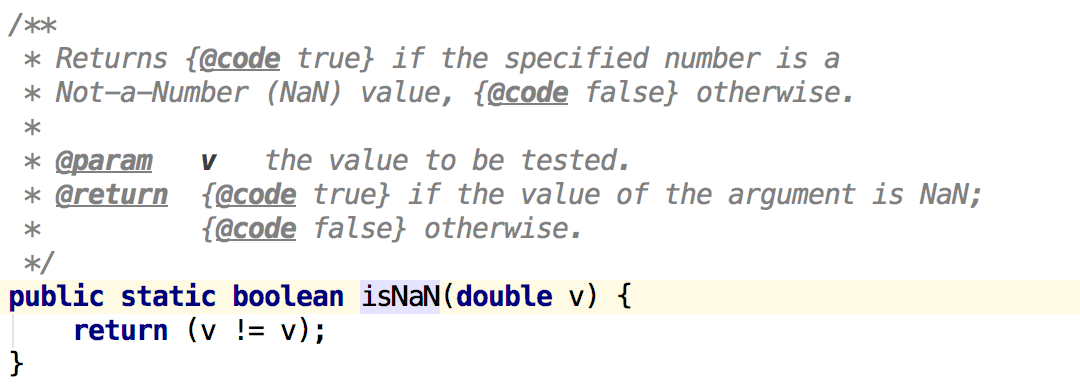java的Math.pow源码看到了这句 if (x != x || y != y)，是什么意思呀？ 5C

public static double pow(double x, double y)
{
// Special cases first.
if (y == 0)
return 1;
if (y == 1)
return x;
if (y == -1)
return 1 / x;
if (x != x || y != y)
return Double.NaN;
.......
}

4个回答x^=y^=x^=y;

Math.pow(x,y)使用注意事项

python中items =[[x, y]for (y, x) in pairs]是什么意思
items是列表nitems =[[x, y]for (y, x) in pairs]是什么意思
c新手请教 x>y?x:y 是什么意思
c新手请教 x>y?x:y 是什么意思

z = (x - y) + y

sin(x)=y,y已知,如何求x?cos(x)=y,y已知,如何求x?

y=x,y=-x图像不垂直的

x或y等于x加y

x && y 先算x还是y ?

x=y?

x=(y
java中 int x=3, y=4;rnx=(y
x^=y
RT
String(x,y,z,a)是什么意思？
String desFile=new String(b4,0,0,bytes2);rnrn说详细点！！！rn谢谢。

x|y表示的是x整除y，y被x整除。 举个栗子：                2|4，意思是4被2整除，即2整除4；                1|3，意思是3被1整除，即1整除3；                 5|15，意思是15被5整除，即5整除15。 我们会发现“|”运算符号左边的数一定小于运算符号右边的数。 这个问题一般会在离散数学中看到。 现在好理解了嘛？...
Canvas->MoveTo(X,Y)是什么意思
void __fastcall TForm1::FormMouseMove(TObject *Sender, TShiftState Shift,rn int X, int Y)rnrn Canvas->MoveTo(X,Y);rnrn其中Canvas是什么啊，做什么用的
fState [x][y] ^= 1 ;是什么意思？

((z < x) ? x : ((z > y) ? y : z))含义是什么？
((z < x) ? x : ((z > y) ? y : z))　含义是什么？
X=5FH,Y=A3H. 则X·Y⊕Y＝？

x的y次方的算法是什么？

java中x^=y^=x^=y交换整形数据Bug

java，已知x!+y!+z!=xyz，求x,y,z
x,y,z是1-9之间的数字。我自己写了一个，不知道哪出错了，求解。。rn[code=Java]rnpackage erfenfaqiujie;rnrnpublic class fsdd rnrn /**rn * @param argsrn */rn public static void main(String[] args) rn // TODO Auto-generated method stubrn // 求 x!+y!+z!=xyz的自然根。rn int x,y,z;rn double x1=1,y1=1,z1=1;rn rn for(int i=100;i<=999;i++)rn x=i/100;rn y=(i/10)%10;rn z=i%10;rn rn for(int j=1;j<=x;j++)rn x1*=j;rn rn for(int k=1;k<=y;k++)rn y1*=k;rn rn for(int l=1;l<=z;l++)rn z1*=l;rn rn rn if(x1+y1+z1==i)rn System.out.println(i);rn rn rn rn rnrnrnrn[/code]rnrn菜鸟求指点。
#define MIN(x,y) x

JAVA画出抛物线y=x*x的图像
JAVA画出抛物线y=x*x的图像，非常靠谱，非常实用
2的x次方=x的y次方，x= ??
2的x次方=x的y次方，x= ??

#define howmany(x, y) ((unsigned int)(((x)+((y)-1)))/(unsigned int)(y))嘛意思？
#define howmany(x, y) ((unsigned int)(((x)+((y)-1)))/(unsigned int)(y))
#define set_s (x,y) strcpy(s[x].name,y);s[x].size=strlen(y); 报错
[code=C/C++]#includern#include rn#define set_s (x,y) strcpy(s[x].name,y);s[x].size=strlen(y);rn#define nmemb 3rnstruct testrnrnchar name;rnint size;rns[nmemb];rnrnvoid main()rnrnrnFILE * stream;rnset_s(0,"Linux!");rnset_s(1,"FreeBSD!");rnset_s(2,"Windows2000.");rnstream=fopen("c:\\a.txt","w");rnfwrite(s,sizeof(struct test),nmemb,stream);rnfclose(stream);rnrn[/code]rn\f.cpp(15) : error C2065: 'x' : undeclared identifierrn'y' : undeclared identifierrn\f.cpp(15) : error C2143: syntax error : missing ';' before ''rn\f.cpp(16) : error C2143: syntax error : missing ';' before ''rn\f.cpp(17) : error C2143: syntax error : missing ';' before ''rnrn说set_s(0,"Linux!");的xy没有定义，怎么整，谢谢~~

x的y次方
x的y次方
Python(x,y)的下载安装
1.下载 下载地址：ftp://ftp.ntua.gr/pub/devel/pythonxy/ 访问该地址，选择需要的版本，点击下载。我安装的版本是2.7.2.3. 2.安装 双击下好的应用程序，接下来选择full,全部安装，等待安装完成就行了。安装中途360可能会弹出“有程序正在修改系统变量”之类的，这是Python(x,y)集成了Python的环境，安装的时候会修改系统变量里...
Python(x,y）的安装
Python(x,y）的安装 下载的网址为：http://pythonxy.connectmv.com/ 安装完成后，界面如图所示： Shortcuts：启动各种应用程序 Documentation：打开各个软件包的文档 About：查看所安装的程序库的版本信息 点击下图中画红圈处 输入“Python”和“print ‘hello Python’”，出现如下图所示界面，Pyt...
gotoxy（x，y）；
#include rnvoid main()rnrn int x=10,y=10;rn gotoxy(x,y);rnrnrnrn在vc下出现error C2065: 'gotoxy' : undeclared identifierrn？？？？？？？

X的Y次方，包括小数，如rn0.5^0.7=0.61557

# define MIN(x, y) ((x) > (y) ? (y) : (x)) 宏展开后还带最外边的小括号吗？
# define MIN(x, y) ((x) > (y) ? (y) : (x))rnrn是（x > y ? y : x）还是 x > y ? y : xrn???rn另外：为什么要加个小括号？